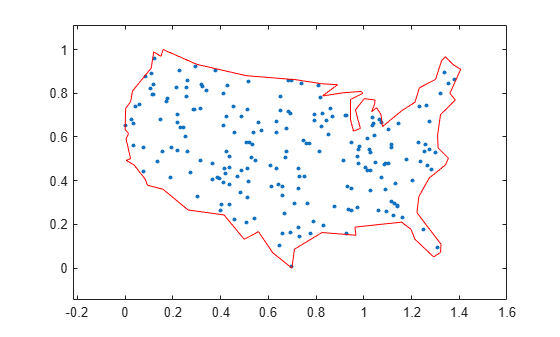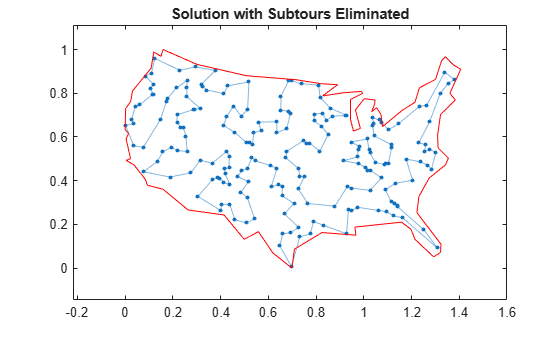## Traveling Salesman Problem: Problem-Based

This example shows how to use binary integer programming to solve the classic traveling salesman problem. This problem involves finding the shortest closed tour (path) through a set of stops (cities). In this case there are 200 stops, but you can easily change the `nStops` variable to get a different problem size. You'll solve the initial problem and see that the solution has subtours. This means the optimal solution found doesn't give one continuous path through all the points, but instead has several disconnected loops. You'll then use an iterative process of determining the subtours, adding constraints, and rerunning the optimization until the subtours are eliminated.

For the solver-based approach to this problem, see Traveling Salesman Problem: Solver-Based.

### Draw the Map and Stops

Generate random stops inside a crude polygonal representation of the continental U.S.

```figure; load('usborder.mat','x','y','xx','yy'); rng(3,'twister') % makes a plot with stops in Maine & Florida, and is reproducible nStops = 200; % you can use any number, but the problem size scales as N^2 stopsLon = zeros(nStops,1); % allocate x-coordinates of nStops stopsLat = stopsLon; % allocate y-coordinates n = 1; while (n <= nStops) xp = rand*1.5; yp = rand; if inpolygon(xp,yp,x,y) % test if inside the border stopsLon(n) = xp; stopsLat(n) = yp; n = n+1; end end plot(x,y,'Color','red'); % draw the outside border hold on % Add the stops to the map plot(stopsLon,stopsLat,'*b') hold off```### Problem Formulation

Formulate the traveling salesman problem for integer linear programming as follows:

• Generate all possible trips, meaning all distinct pairs of stops.

• Calculate the distance for each trip.

• The cost function to minimize is the sum of the trip distances for each trip in the tour.

• The decision variables are binary, and associated with each trip, where each 1 represents a trip that exists on the tour, and each 0 represents a trip that is not on the tour.

• To ensure that the tour includes every stop, include the linear constraint that each stop is on exactly two trips. This means one arrival and one departure from the stop.

### Calculate Distances Between Points

Because there are 200 stops, there are 19,900 trips, meaning 19,900 binary variables (# variables = 200 choose 2).

Generate all the trips, meaning all pairs of stops.

`idxs = nchoosek(1:nStops,2);`

Calculate all the trip distances, assuming that the earth is flat in order to use the Pythagorean rule.

```dist = hypot(stopsLat(idxs(:,1)) - stopsLat(idxs(:,2)), ... stopsLon(idxs(:,1)) - stopsLon(idxs(:,2))); lendist = length(dist);```

With this definition of the `dist` vector, the length of a tour is

`dist'*trips`

where `trips` is the binary vector representing the trips that the solution takes. This is the distance of a tour that you try to minimize.

### Create Variables and Problem

Create a problem and binary variables.

```tsp = optimproblem; trips = optimvar('trips',lendist,1,'Type','integer','LowerBound',0,'UpperBound',1);```

Include the objective function in the problem.

`tsp.Objective = dist'*trips;`

### Equality Constraints

The problem has two types of equality constraints. The first enforces that there must be 200 trips total. The second enforces that each stop must have two trips attached to it (there must be a trip to each stop and a trip departing each stop).

Specify the first type of equality constraint, that you must have `nStops` trips, and include it in the problem.

```constrips = sum(trips) == nStops; tsp.Constraints.constrips = constrips;```

To specify the second type of equality constraint, that there needs to be two trips attached to each stop, find the trips for each stop and add up the number of trips for that stop. Look at trips that both start and end at that stop.

```constr2trips = optimconstr(nStops,1); for stops = 1:nStops whichIdxs = (idxs == stops); whichIdxs = any(whichIdxs,2); % start or end at stops constr2trips(stops) = sum(trips(whichIdxs)) == 2; end tsp.Constraints.constr2trips = constr2trips;```

### Solve the Initial Problem

The problem is ready to be solved. To suppress iterative output, turn off the default display.

```opts = optimoptions('intlinprog','Display','off'); tspsol = solve(tsp,'options',opts)```
```tspsol = struct with fields: trips: [19900×1 double] ```

### Visualize the Solution

```hold on segments = find(tspsol.trips); % Get indices of lines on optimal path lh = zeros(nStops,1); % Use to store handles to lines on plot lh = updateSalesmanPlot(lh,tspsol.trips,idxs,stopsLon,stopsLat); title('Solution with Subtours');```As can be seen on the map, the solution has several subtours. The constraints specified so far do not prevent these subtours from happening. In order to prevent any possible subtour from happening, you would need an incredibly large number of inequality constraints.

### Subtour Constraints

Because you can't add all of the subtour constraints, take an iterative approach. Detect the subtours in the current solution, then add inequality constraints to prevent those particular subtours from happening. By doing this, you find a suitable tour in a few iterations.

Eliminate subtours with inequality constraints. An example of how this works is if you have five points in a subtour, then you have five lines connecting those points to create the subtour. Eliminate this subtour by implementing an inequality constraint to say there must be less than or equal to four lines between these five points.

Even more, find all lines between these five points, and constrain the solution not to have more than four of these lines present. This is a correct constraint because if five or more of the lines existed in a solution, then the solution would have a subtour (a graph with $n$ nodes and $n$ edges always contains a cycle).

The `detectSubtours` function analyzes the solution and returns a cell array of vectors. Each vector in the cell array contains the stops involved in that particular subtour.

```tours = detectSubtours(tspsol.trips,idxs); numtours = length(tours); % number of subtours fprintf('# of subtours: %d\n',numtours);```
```# of subtours: 27 ```

Include the linear inequality constraints to eliminate subtours, and repeatedly call the solver, until just one subtour remains.

```% Index of added constraints for subtours k = 1; while numtours > 1 % repeat until there is just one subtour % Add the subtour constraints for ii = 1:numtours subTourIdx = tours{ii}; % Extract the current subtour % The next lines find all of the variables associated with the % particular subtour, then add an inequality constraint to prohibit % that subtour and all subtours that use those stops. variations = nchoosek(1:length(subTourIdx),2); a = false(length(idxs),1); for jj = 1:length(variations) whichVar = (sum(idxs==subTourIdx(variations(jj,1)),2)) & ... (sum(idxs==subTourIdx(variations(jj,2)),2)); a = a | whichVar; end tsp.Constraints.(sprintf('subtourconstr%i',k)) = sum(trips(a)) <= length(subTourIdx)-1; k = k + 1; end % Try to optimize again [tspsol,fval,exitflag,output] = solve(tsp,'options',opts); % Visualize result lh = updateSalesmanPlot(lh,tspsol.trips,idxs,stopsLon,stopsLat); % How many subtours this time? tours = detectSubtours(tspsol.trips,idxs); numtours = length(tours); % number of subtours fprintf('# of subtours: %d\n',numtours); end```
```# of subtours: 20 ```
```# of subtours: 7 ```
```# of subtours: 9 ```
```# of subtours: 9 ```
```# of subtours: 3 ```
```# of subtours: 2 ```
```# of subtours: 7 ```
```# of subtours: 2 ```
```# of subtours: 1 ```
```title('Solution with Subtours Eliminated'); hold off```### Solution Quality

The solution represents a feasible tour, because it is a single closed loop. But is it a minimal-cost tour? One way to find out is to examine the output structure.

`disp(output.absolutegap)`
``` 0 ```

The smallness of the absolute gap implies that the solution is either optimal or has a total length that is close to optimal.

Watch now